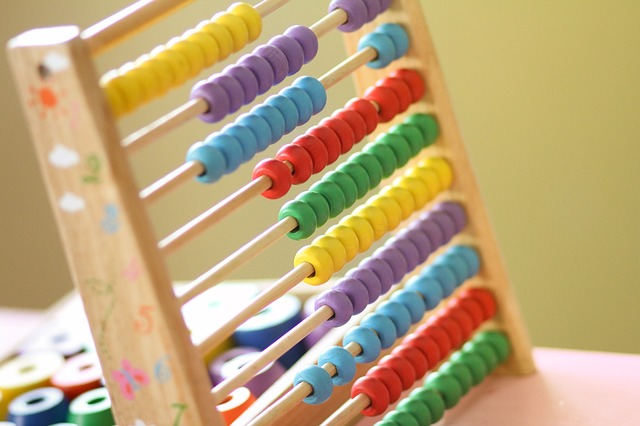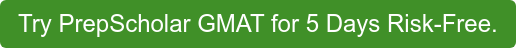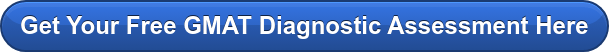# GMAT Remainder Problems: 3 Key TipsIf you’re like me, you probably haven’t thought about remainders in over ten years, when you first learned about long division in elementary school. Remember those lessons on what we call the number that’s left over in a division problem? Yeah, that number’s called a remainder.

And guess what? Remainders show up a lot on the GMAT. Of course, the remainder problems you’ll encounter on the GMAT are much different than the ones you worked on when you were ten years old. GMAT remainder questions can often be quite tricky, but don’t worry! In this guide, I’ll give you a comprehensive overview of GMAT remainder problems so that you feel ready to solve them when you see them on test day.

First, I’ll walk you through a brief refresher course on remainders. Next, I’ll offer some tips for solving GMAT remainder questions. Finally, I’ll provide some sample GMAT remainder problems and explanations for you to study.

## What Is A Remainder?

If you’re a bit fuzzy on what a remainder actually is, don’t sweat it. Many GMAT test takers haven’t worked with remainders in many years. Let’s start by going over the basics of what a remainder is and how you’ll see them tested on the GMAT.

Think back to that fourth grade math class you took a long time ago. Let’s review the specific terminology we use to talk about division:

Not sure how or what to study? Confused by how to improve your score in the shortest time possible? We've created the only Online GMAT Prep Program that identifies your strengths and weaknesses, customizes a study plan, coaches you through lessons and quizzes, and adapts your study plan as you improve.

We believe PrepScholar GMAT is the best GMAT prep program available, especially if you find it hard to organize your study schedule and don't want to spend a ton of money on the other companies' one-size-fits-all study plans.• When you divide \$x\$ by \$y\$, it’s the same as the fraction “\$x/y\$.”
• If we were dividing 6 by 3 (or, \$6/3\$), 6, the term we’re dividing by something else, would be the “dividend.”
• 3, the number that’s doing the dividing, is the “divisor.”
• In the case of the simple division problem \$6/3\$, 2 is our answer, or our “quotient.”

But, as I’m sure you know, not all division problems are as nice and neat as \$6/3\$. That’s where remainders come in.

Simply put, the remainder is the fraction part (or what “remains”) when you divide two numbers that don’t result in a whole number quotient.

For instance, when you divide \$8/3\$, the remainder is 2. When you divide 8 by 3, you have two sets of 3, with 2 leftover.

An easy way to think about remainders is to think about them as mixed numbers. For instance, the fraction \$8/3\$ is the same thing as the mixed number \$2 2/3\$. \$2/3\$ represents our remainder. We express it as \$2/3\$ because we have 2 parts left out of the 3 parts we need to make a whole number. The denominator will always be the same as the divisor.

Now that we’ve reviewed the basics, let’s move on to some more complicated, GMAT-style remainder math. Let’s say that we’re dividing our dividend \$a\$ by our divisor \$b\$ to yield our whole number quotient \$c\$ and our remainder \$d\$. That translates into the following equation:

\$\$a/b=c+d/b\$\$

For instance, in the simple problem we worked through before:

• \$a\$: 8 (dividend)
• \$b\$: 3 (divisor)
• \$c\$: 2 (quotient)
• \$d\$: 2 (remainder)

It’s worth memorizing this basic remainder equation – it’ll come up fairly often on the GMAT.

Remember, remainders can also be expressed as decimals. For instance, the remainder \$2/3\$ could be represented as .66 (repeating). That’s what you’ll likely see when you’re using a calculator (e.g., \$8/3=2.66666\$).

GMAT remainder problems are obviously more complex than what we’ve gone over in this section. For the GMAT, you’ll be asked to apply your knowledge of the basic relationship between dividend, divisor, quotient, and remainder to solve moderate to advanced algebraic equations.

As with all GMAT quant questions, we’ll have to rely on more than just our basic remainders knowledge to get the right answer. In the next section, I’ll give you some tips on solving GMAT remainder questions and then walk you through solving four sample questions.

## Tips for Solving GMAT Remainder Questions

While GMAT remainder problems can be quite complicated, there are some things you can to do to more easily solve the problems you encounter. Keep in mind these tips as you’re working on GMAT remainder problems.

### #1: Memorize the Remainder Relationship Formula

In the previous section, I gave you the formula for finding the remainder of a division equation:

\$a/b=c+d/b\$, where \$a\$ = dividend; \$b\$ = divisor, \$c\$ = quotient, and \$d\$ = remainder.

Knowing this equation is the key to answering remainder questions on the GMAT. You should also be comfortable moving around the variables in the equation, so that you understand the other relationships this equation yields such as:

\$\$a=cb+d\$\$

Being able to quickly recall the remainder equation and manipulate it in different ways by moving the variables on either side of the equal sign will really help you on the GMAT. You’ll be able to correctly plug-in the different numbers and formulas the GMAT throws at you so that you can figure out exactly what you need to solve.

### #2: Plug-In Numbers for Variables

A great way to work out tricky remainder questions is to plug-in numbers for variables into your GMAT remainders equation. While this strategy won’t work all the time, plugging in numbers can be helpful if you’re stuck and unsure how to get to an answer. Let’s look at a sample question to see how this works:

What is the remainder when \$x\$ is divided by \$3\$, if the sum of the digits of \$x\$ is 5?

A. 2
B. 3
C. 4
D. 5

E. 6

Want to improve your GMAT score by 60 points?

We have the industry's leading GMAT prep program. Built by Harvard, MIT, Stanford, and Wharton alumni and GMAT 99th percentile scorers, the program learns your strengths and weaknesses and customizes a curriculum so you get the most effective prep possible.That means we can plug in a number for \$x\$ that has digits that add together to equal five. For instance, we can use the number 14, because \$1 + 4 = 5\$. If we divide \$14/3\$, we find out that the remainder is 2. Let’s keep testing this theory. If we plug in the number 50 for \$x\$ (because \$5 + 0 = 5\$), we get the equation \$50/3\$, which equals 16 remainder 2. We can test this one more time with \$23\$ (\$2 + 3 = 5\$). If we divide \$23/3\$, we get 7 remainder 2. That tells us that the answer is A: 2.

Plugging in numbers doesn’t always work, particularly if you’ve got a number of different unknown variables. However, if you’ve got a relatively small set of potential numbers (e.g., numbers whose digits add up to five), you can plug in numbers to test for the correct answer.

### #3: Learn Remainder Shortcuts

There are several nifty remainder “shortcuts” that you should keep in mind when you’re working on GMAT remainder questions. These shortcuts will save you time so you don’t have to completely write out calculations.

The possible remainders when a number is divided by a divisor \$b\$ can range from 0 to one less than \$b\$. For example, if \$b\$ = 5, the possible remainders are then from 0 – 4 (which is one less than 5). If \$b\$ = 10, the possible remainders range from 0 – 9 (which is one less than 10).

If a number is divided by 10, its remainder is the last digit of that number. If you divide it by 100, its remainder is the last two digits of that number, and so on. For, example 49 divided by 10 equals 4 with a remainder of 9.

You can take the decimal portion of the quotient and multiply it by the divisor to get the remainder. For example, if we know \$9/5=1.8\$ we can multiply .8 by the divisor 5, which gives us the remainder, 4. Keep in mind that you won’t have a calculator on the GMAT, though, so this tip may or may not save you time.

## GMAT Remainder Problem Examples

Now that we’ve learned some tips for solving remainder questions, let’s see them in action. In these GMAT remainder problems, I’ll walk you through how to solve each question using the equations, tips, and tricks we’ve discussed earlier in the article.

### Problem Solving Remainder Sample Question

When positive integer \$x\$ is divided by positive integer \$y\$, the remainder is 9. If \$x/y\$ = 96.12, what is the value of \$y\$?

A. 96
B. 75
C. 48
D. 25

E. 12

Let’s start with this question by plugging what we know into our remainder equation. In this case, we know the following:

\$x\$/\$y\$ = \$q\$ + 9, where \$q\$ is the quotient (which is unknown), \$x\$ is the dividend (unknown), and \$y\$ is the divisor (also unknown).

From the question, we also know that \$x/y = 96.12\$. Remember how we talked about decimals earlier? When we’re giving an answer to a division problem that has a decimal in it, the whole number (in this case, 96) is the quotient and the decimal is the remainder. So, in this case, we express our answer as 96 + 0.12, with 96 as the quotient and 0.12 is the remainder.

We can also express our remainder as \$\remainder/\divisor\$, which gives us the equation:

Want to Identify YOUR GMAT Strengths and Weaknesses?

Our proprietary GMAT Diagnostic Assessment creates a customized study plan for you that takes you from registration all the way to test day! It is included with every account and proven to significantly maximize your score.

Get your personalized assessment as part of your 5 day risk-free trial now:\$\$x/y = q + 9/y\$\$

Since we now have two values for \$x/y\$, we can set them equal to each other, which yields:

\$\$96 + 9/y = 96.12\$\$

We can solve this equation through by subtracting 96 from the left side of the equation so we get:

\$\$9/y = .12\$\$

That leaves us with \$9/y = 0.12\$, or \$y = 75\$.

### Data Sufficiency Remainder Sample Question

What is the tens digit of positive integer \$x\$?

1. \$x\$ divided by 100 has a remainder of 30.
2. \$x\$ divided by 110 has a remainder of 30.
A. Statement (1) ALONE is sufficient, but statement (2) alone is not sufficient.
B. Statement (2) ALONE is sufficient, but statement (1) alone is not sufficient.
C. BOTH statements TOGETHER are sufficient, but NEITHER statement ALONE is sufficient.
D. EACH statement ALONE is sufficient.

E. Statements (1) and (2) TOGETHER are NOT sufficient.

When we approach data sufficiency questions, we always want to solve each statement alone before looking at them together. Keeping that in mind, let’s look at statement (1) first.

Remember, we’re trying to figure out if we can determine the tens digit of a positive integer.

Statement (1) tells us that, when our positive integer \$x\$ is divided by 100, it has a remainder of 30.

This statement is a good example of something that you can plug a number into. For this, I’m going to try dividing different numbers by 100. I’ll start with 100. When you divide 100 by 100, you don’t get a remainder. When you divide 110 by 100, you get a remainder of 10. When you divide 120 by 100, you get a remainder of 20. When you divide 130 by 100, you get a remainder of 30.

You can also use the remainder shortcut that, if any number is divided by 100, its remainder is the last two digits of that number. That tells us that tens digit of our number has to be 3. This statement is sufficient.

Now let’s try statement (2). Remember, we want to look at each statement by itself first.

We can try plugging in numbers for statement (2). Using the same method of plugging in every number from 100 to 300 counting by tens, I find that if I divide 140 by 110, my quotient is 1 remainder 30. However, if I divide 250 by 110, I get a quotient of 2 remainder 30.

In this case, I have two different numbers that both yield me a remainder of 30. That means statement (2) is not sufficient.

I can’t use statement (1) and statement (2) together because they are mutually exclusive. Therefore, my answer is A.

## Review: GMAT Remainders

While remainders may seem like elementary math you haven’t touched in years and don’t need to remember, remainder questions will often appear on the GMAT.

The most important step in solving GMAT remainder problems is to memorize the remainders relationships equations. However, plugging-in numbers and recognizing patterns can also help you solve these tricky questions.

## What’s Next?

Are you confident in solving remainders questions now? Looking to move onto a new GMAT quant challenge? We have in-depth guides on many of the math concepts you’ll see on the GMAT. Check out our guides to GMAT geometry and GMAT rate problems to boost your knowledge on two other commonly tested GMAT concepts.

If you’re looking for a more generalized overview of the GMAT quant section, our GMAT quant guide will give you a solid overview of the content of the GMAT quant section, while our GMAT quant practice guide will help you better understand how and what to practice to ace the quant section.

Looking to completely change it up? If you want to focus on verbal instead, our in-depth guide to the GMAT verbal section will give you a great overview of the GMAT verbal section, while also suggesting resources you can use to practice.